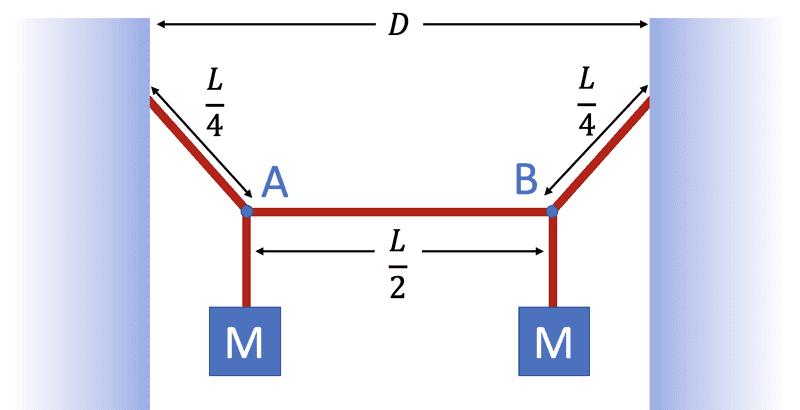# Waves- sending a pulse across a weighted line

• Eaoke3

#### Eaoke3

Homework Statement
A light string with a mass of 10.2 g and a length L=3.40 m has its ends tied to two walls that are separated by the distance D=1.85 m. Two objects, each with a mass M=1.83 kg, are suspended from the string, as shown in the figure below.

If a wave pulse is sent from point A, how long (in milliseconds) does it take for it to travel to point B?
Relevant Equations
I was trying to use f=1/2LSqrt(Ftension/u)
or there is also v=sqrt(Ftension/u)

lambda=2L/m

v= f/lambdaHere is a picture of the problem:

I honestly am pretty lost, I'm not looking for an answer, more so an idea to get me started. But here is what I was thinking:

In the equation above I was trying to use:
For U I am unsure how to incorporate the weight of the blocks into the u, so I am unsure how to calculate that.

For the Force of tension- I am unsure if you are able to solve for this, as we don’t know any of the angles.

I would then use that to plug into the second equation to find V, then divide that by (L/2) <---the distance.

A light string with a mass of 10.210.2 g
That number has too many decimal points

... we don’t know any of the angles.
Of course you do. A bit of calculation is required, sure, but you DO have them.

I corrected it, thank you :- ).

Ohh, well using the L and its corresponding dividing value, I would be able to obtain lengths, but from there I am unsure of how to apply those numbers into anything useful.

I can see that I have different values and variables, but I really think I am missing a big conceptual picture of how to put them all together.

I corrected it, thank you :- ).

Ohh, well using the L and its corresponding dividing value, I would be able to obtain lengths, but from there I am unsure of how to apply those numbers into anything useful.
Can you not see how to use trig to get the angles? I don't know if they are needed to solve the problem but if they are you can get them.